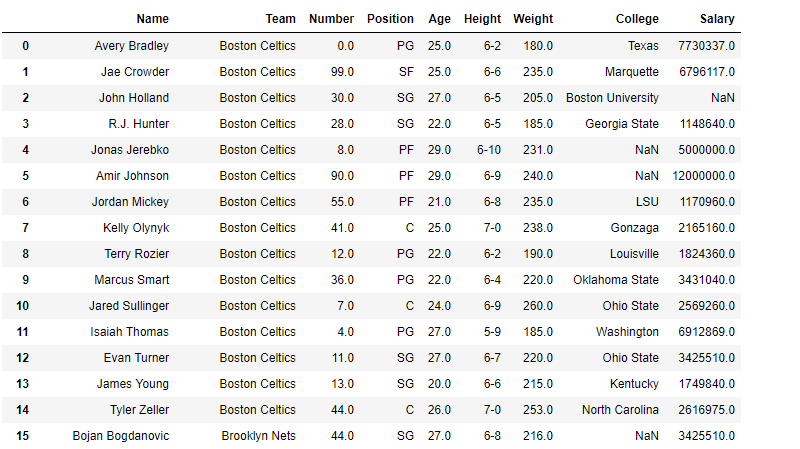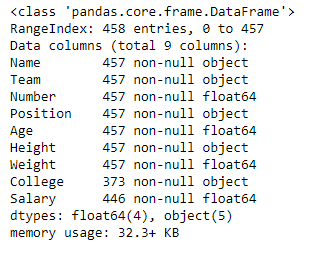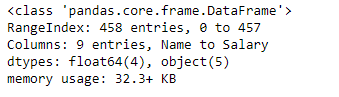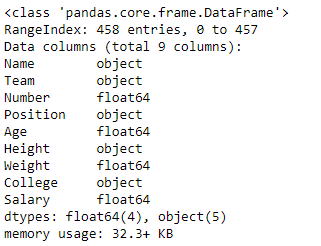Open in App
Not now

# Python | Pandas dataframe.info()

• Last Updated : 25 Aug, 2021

Python is a great language for doing data analysis, primarily because of the fantastic ecosystem of data-centric python packages. Pandas is one of those packages and makes importing and analyzing data much easier.
Pandas dataframe.info() function is used to get a concise summary of the dataframe. It comes really handy when doing exploratory analysis of the data. To get a quick overview of the dataset we use the dataframe.info() function.

Syntax: DataFrame.info(verbose=None, buf=None, max_cols=None, memory_usage=None, null_counts=None)
Parameters :
verbose : Whether to print the full summary. None follows the display.max_info_columns setting. True or False overrides the display.max_info_columns setting.
buf : writable buffer, defaults to sys.stdout
max_cols : Determines whether full summary or short summary is printed. None follows the display.max_info_columns setting.
memory_usage : Specifies whether total memory usage of the DataFrame elements (including index) should be displayed. None follows the display.memory_usage setting. True or False overrides the display.memory_usage setting. A value of ‘deep’ is equivalent of True, with deep introspection. Memory usage is shown in human-readable units (base-2 representation).
null_counts : Whether to show the non-null counts. If None, then only show if the frame is smaller than max_info_rows and max_info_columns. If True, always show counts. If False, never show counts.

Example #1: Use info() function to print full summary of the dataframe.

## Python3

 `# importing pandas as pd``import` `pandas as pd` `# Creating the dataframe``df ``=` `pd.read_csv(``"nba.csv"``)` `# Print the dataframe``df`Let’s print the full summary of the dataframe.

## Python3

 `# to print the full summary``df.info()`

Output :As we can see in the output, the summary includes list of all columns with their data types and the number of non-null values in each column. we also have the value of rangeindex provided for the index axis.

Example #2: Use info() function to print a short summary of the dataframe
Note : In order to print the short summary, we can use the verbose parameter and set it to False.

## Python3

 `# importing pandas as pd``import` `pandas as pd` `# Creating the dataframe``df ``=` `pd.read_csv(``"nba.csv"``)` `# Print the short summary of the``# dataframe by setting verbose = False``df.info(verbose ``=` `False``)`

Output :As, we can see in the output, the summary is very crisp and short. It is helpful when we have 1000s of attributes in dataframe.

Example #3: Use info() function to print a full summary of the dataframe and exclude the null-counts.
Note : In order to print the full summary, with null-counts excluded, we can use null-counts parameter and set it to be false.

## Python3

 `# importing pandas as pd``import` `pandas as pd` `# Creating the dataframe``df ``=` `pd.read_csv(``"nba.csv"``)` `# Print the full summary of the dataframe``# with null count excluded``df.info(verbose ``=` `True``, null_counts ``=` `False``)`

Output :As, we can see in the output, the summary is full but null-counts are excluded.

My Personal Notes arrow_drop_up